## Using DataCamp reduces anxiety about learning R!

I used DataCamp‘s excellent Introduction to R as Essential Prior Independent Study and found it made people a bit less worried about a term of R!I have a lot of fun teaching first year biology undergraduates but there are a few challenges in teaching data skills when they are not (perceived as) a student’s core discipline but instead required to carry out research within it. At this early stage in their higher education, Biologists can be surprised by the amount of their degree devoted to data analysis, reporting and presentation.

In my introductory lecture I use polling software to get responses from my students to:As you can see, my students don’t mind making their feelings clear!

Those are the results from the last two years – if anything, this year’s students are more sure they won’t enjoy it! I suspect this is not the result my colleagues teaching Genetics, Evolution, Cell Biology or Development would get if they asked the same. And understandably, I think.

This year I set Essential Prior Independent Study using the ability to set “Assignments” for a team in my DataCamp‘ classroom. I had them do only the first two chapters (Intro to basics and Vectors) of Introduction to R. Last year I suggested DataCamp as an optional  activity and used part of it in an introductory workshop.

I was delighted to see that well over half the class of 256 students had started or completed the assignments before the lecture despite the assignment deadline still being a day away. And there was more……..when I asked how they felt about R, they were more positive than last year:How good is that? Many ‘Seems Ok’ and ‘Undecided’ and more students excited than terrified is a win!

Well done them!# Help! The same annotations go on every facet!(with thanks to a student for sending me her attempt).

This is a question I get fairly often and the answer is not straightforward especially for those that are relatively new to R and ggplot2.

In this post, I will show you how to add different annotations to each facet. More like this:This is useful in its own right but can also help you understand `ggplot` better.

I will assume you have R Studio installed and have at least a little experience with it but I’m aiming to make this do-able for novices. I’ll also assume you’ve analysed your data so you know what annotations you want to add.

Faceting is a very useful feature of `ggplot` which allows you to efficiently plot subsets of your data next to each other.
In this example the data are the wing lengths for males and females of two imaginary species of butterfly in two regions, north and south. Some of the results of a statistical analysis are shown with annotation.

## 1. Preparation

The first thing you want to do is make a folder on your computer where your code and the data for plotting will live. This is your working directory.
Now get a copy of the data by saving this file the folder you just made.

## 2. Start in RStudio

Start R Studio and set your working directory to the folder you created: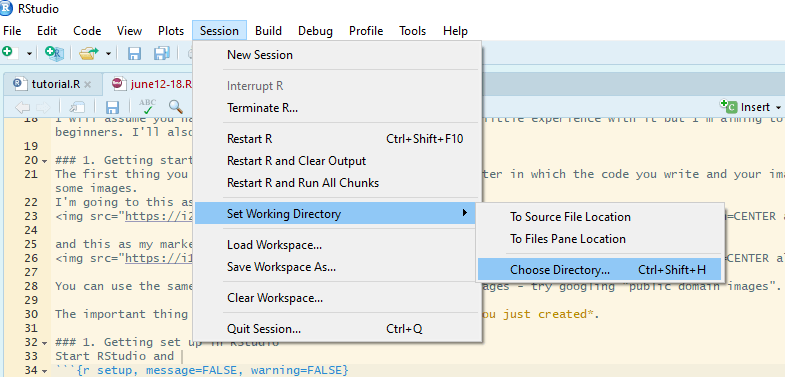Now start a new script file: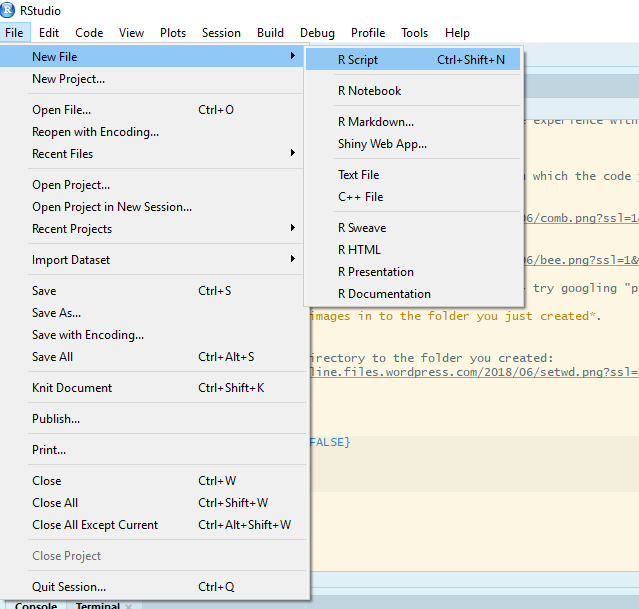and save it as figure.R or similar.

## 3. Load packages

Make the packages you need available for use in this R Studio session by adding this code to your script and running it.

``````# package loading
library(ggplot2)
library(Rmisc)
``````

## 4. Read the data in to R

The data are in a plain text file where the first row gives the column names. It can be read in to a dataframe with the `read.table()` command:

``````butter <- read.table("butterflies.txt", header = TRUE)
``````

This each row in this data set is an individual butterfly and the columns are four variables:

• `winglen` the wing length (in millimeters) of an individual
• `spp` its species, one of “F.concocti” or “F.flappa”
• `sex` its sex, one of “female” or “male”
• `region` where it is from, one of “North” or “South”

## 5. Summarise the data

Our plot has the means and standard errors for each group and this requires us to summarize over the replicates which we can do with the `summarySE()` function:

``````buttersum <- summarySE(data = butter, measurevar = "winglen",
groupvars = c("spp", "sex", "region"))
buttersum
``````
```##          spp    sex region  N  winglen       sd        se       ci
## 1 F.concocti female  North 10 25.93591 4.303011 1.3607315 3.078189
## 2 F.concocti female  South 10 31.37000 4.275265 1.3519574 3.058340
## 3 F.concocti   male  North 10 23.22876 4.250612 1.3441617 3.040705
## 4 F.concocti   male  South 10 24.97000 4.957609 1.5677337 3.546460
## 5   F.flappa female  North 10 33.18389 4.286312 1.3554509 3.066243
## 6   F.flappa female  South 10 24.67000 3.270423 1.0341986 2.339520
## 7   F.flappa   male  North 10 24.46586 5.492053 1.7367398 3.928778
## 8   F.flappa   male  South 10 23.45000 3.012290 0.9525696 2.154862
```

A group is a species-sex-region combination.

## 6. Plot

We have four variables to plot. Three are explanatory: species, sex and region. We map one of the explanatory variables to the x-axis, one to different colours and one to the facets.

To plot North and South on separate facets, we tell `facet_grid()` to plot everything else (`.`) for each region:

``````ggplot(data = buttersum, aes(x = spp, y = winglen)) +
geom_point(aes(colour = sex), position = position_dodge(width = 1)) +
geom_errorbar(aes(colour = sex, ymin = winglen - se, ymax = winglen + se),
width = .2, position = position_dodge(width = 1)) +
ylim(0, 40) +
facet_grid(. ~ region)
``````## Build understanding

This section will help you understand why facet annotations are done as they are but you can go straight to 7. Create a dataframe for the annotation information if you just want the code.

We plan to facet by region but in order to understand better, it is useful to first plot just one region. We can subset the data to achieve that:

#### a) Subset

``````# subset the northern region
butterN <- butter[butter\$region == "North",]
``````

#### b) Summarise data subset for plotting

``````butterNsum <- summarySE(data = butterN, measurevar = "winglen",
groupvars = c("spp", "sex"))
butterNsum
``````
```##          spp    sex  N  winglen       sd       se       ci
## 1 F.concocti female 10 25.93591 4.303011 1.360732 3.078189
## 2 F.concocti   male 10 23.22876 4.250612 1.344162 3.040705
## 3   F.flappa female 10 33.18389 4.286312 1.355451 3.066243
## 4   F.flappa   male 10 24.46586 5.492053 1.736740 3.928778
```

#### c) Plot subset

Since we are dealing only with data from the North, we have just three variables to plot. We map one of the explanatory variables to the x-axis and the other to different colours:

``````ggplot(data = butterNsum, aes(x = spp, y = winglen, colour = sex)) +
geom_point(position = position_dodge(width = 1)) +
geom_errorbar(aes(ymin = winglen - se, ymax = winglen + se),
width = .2, position = position_dodge(width = 1)) +
ylim(0, 40)
``````• `data = butterNsum` tells ggplot which dataframe to plot (the summary)
• `aes(x = spp, y = winglen, colour = sex)` the “aesthetic mappings” specify where to put each variable. Aesthetic mappings given in the `ggplot()` statement will apply to every “layer” in the plot unless otherwise specified.
• `geom_point()` the first “layer” adds points
• `position = position_dodge(width = 1)` indicates female and male means should be plotted side-by-side for each species not on top on each other
• `geom_errorbar()` the second layer adds the error bars. These must also be position dodged so they appear on the points.
• `aes(ymin = winglen - se, ymax = winglen + se)` The error bars need new aesthetic mappings because they are not at winglen (the mean in the summary) but at the mean – the standard error and the mean + the standard error. Since all of that information is inside butterNsum, we do not need to give the data argument again.

#### d) Annotate this plot (i)

The annotation is composed of three lines – or segments – and some text. Each segment has a start (x, y) and an end (xend, yend) which we need to specify. The text is centered on its (x, y)

The x-axis has two categories which have the internal coding of 1 and 2. We want the annotation to start a bit before 2 and finish a bit after 2.

Note that position_dodge() units are twice the category axis units in this example.

``````ggplot(data = butterNsum, aes(x = spp, y = winglen, colour = sex)) +
geom_point(position = position_dodge(width = 1)) +
geom_errorbar(aes(ymin = winglen - se, ymax = winglen + se),
width = .2, position = position_dodge(width = 1)) +
geom_text(x = 2,  y = 38,
label = "***",
colour = "black") +
geom_segment(x = 1.75, xend = 1.75,
y = 36, yend = 37,
colour = "black") +
geom_segment(x = 2.25, xend = 2.25,
y = 36, yend = 37,
colour = "black") +
geom_segment(x = 1.75, xend = 2.25,
y = 37, yend = 37,
colour = "black") +
ylim(0, 40)
``````#### e) Annotate this plot (ii)

Instead of hard coding the co-ordinates into the plot, we could have put them in a dataframe with a column for each x or y as follows:``````anno <- data.frame(x1 = 1.75, x2 = 2.25, y1 = 36, y2 = 37, xstar = 2, ystar = 38, lab = "***")
anno
``````
```##     x1   x2 y1 y2 xstar ystar lab
## 1 1.75 2.25 36 37     2    38 ***
```

Then give a dataframe argument to `geom_segment()` and `geom_text()` and the aesthetic mappings for that dataframe. We also need to move the colour mapping from the `ggplot()` statement to the `geom_point()` and `geom_errorbar()`.

This is because the mappings applied in the `ggplot()` will apply to every layer unless otherwise specified and if the colour mapping stays there, `geom_segment()` and `geom_text()` will try to find the variable ‘sex’ in the anno dataframe.

``````ggplot(data = butterNsum, aes(x = spp, y = winglen)) +
geom_point(aes(colour = sex), position = position_dodge(width = 1)) +
geom_errorbar(aes(colour = sex, ymin = winglen - se, ymax = winglen + se),
width = .2, position = position_dodge(width = 1)) +
ylim(0, 40) +
geom_text(data = anno, aes(x = xstar,  y = ystar, label = lab)) +
geom_segment(data = anno, aes(x = x1, xend = x1,
y = y1, yend = y2),
colour = "black") +
geom_segment(data = anno, aes(x = x2, xend = x2,
y = y1, yend = y2),
colour = "black") +
geom_segment(data = anno, aes(x = x1, xend = x2,
y = y2, yend = y2),
colour = "black")
``````## 7. Create a dataframe for the annotation information

The easiest way to annotate for each facet separately is to create a dataframe with a row for each facet:``````anno <- data.frame(x1 = c(1.75, 0.75), x2 = c(2.25, 1.25),
y1 = c(36, 36), y2 = c(37, 37),
xstar = c(2, 1), ystar = c(38, 38),
lab = c("***", "**"),
region = c("North", "South"))
anno
``````
```##     x1   x2 y1 y2 xstar ystar lab region
## 1 1.75 2.25 36 37     2    38 ***  North
## 2 0.75 1.25 36 37     1    38  **  South
```

## 7. Annotate the plot

Use the annotation dataframe as the value for the data argument in `geom_segment()` and `geom_text()`
New aesthetic mappings will be needed too:

``````ggplot(data = buttersum, aes(x = spp, y = winglen)) +
geom_point(aes(colour = sex), position = position_dodge(width = 1)) +
geom_errorbar(aes(colour = sex, ymin = winglen - se, ymax = winglen + se),
width = .2, position = position_dodge(width = 1)) +
ylim(0, 40) +
geom_text(data = anno, aes(x = xstar,  y = ystar, label = lab)) +
geom_segment(data = anno, aes(x = x1, xend = x1,
y = y1, yend = y2),
colour = "black") +
geom_segment(data = anno, aes(x = x2, xend = x2,
y = y1, yend = y2),
colour = "black") +
geom_segment(data = anno, aes(x = x1, xend = x2,
y = y2, yend = y2),
colour = "black")+
facet_grid(. ~ region)
``````Or a little more report friendly:

``````ggplot(data = buttersum, aes(x = spp, y = winglen)) +
geom_point(aes(shape = sex), position = position_dodge(width = 1), size = 2) +
scale_shape_manual(values = c(1, 19), labels = c("Female", "Male") )+
geom_errorbar(aes(group = sex, ymin = winglen - se, ymax = winglen + se),
width = .2, position = position_dodge(width = 1)) +
ylim(0, 40) +
ylab("Wing length (mm)") +
xlab("") +
geom_text(data = anno, aes(x = xstar,  y = ystar, label = lab)) +
geom_segment(data = anno, aes(x = x1, xend = x1,
y = y1, yend = y2),
colour = "black") +
geom_segment(data = anno, aes(x = x2, xend = x2,
y = y1, yend = y2),
colour = "black") +
geom_segment(data = anno, aes(x = x1, xend = x2,
y = y2, yend = y2),
colour = "black")+
facet_grid(. ~ region) +
theme(panel.background = element_rect(fill = "white", colour = "black"),
strip.background = element_rect(fill = "white", colour = "black"),
legend.key = element_blank(),
legend.title = element_blank())
``````If you want to add images to each facet you can use the `ggimage` package. I covered this in a previous blog, Fun and easy R graphs with images
You need to add column to your annotation dataframe.

## Package references

Hope R.M. (2013). Rmisc: Rmisc: Ryan Miscellaneous. R package version 1.5. https://CRAN.R-project.org/package=Rmisc

Wickham H. (2016). ggplot2: Elegant Graphics for Data Analysis. Springer-Verlag New York. ISBN 978-3-319-24277-4

Yu G. (2018). ggimage: Use Image in ‘ggplot2’. R package version 0.1.7. https://CRAN.R-project.org/package=ggimage

R and all it’s packages are free so don’t forget to cite the awesome contributors.
How to cite packages in R

## R Code Anatomy

It’s a challenge for an experienced user to remember what it was like to be totally new to R and come up with explanations that don’t draw on understanding developed subsequently. Terminology with which you have become very familiar is, in fact, jargon. So I asked a novice, Elliot, to explain a piece of code in his own words. Elliot is a 17 year old student half way through a BTEC Level 3 Extended Diploma in Science which is a qualification which provides access to Higher Education in the UK.

I have annotated with the jargon. In doing so I realised that the c() function is perhaps not the best example but that’s for another post.
R code Anatomy – a nice big image

## RStudio Anatomy

RStudio makes R easier to use. It includes a code editor, debugging & visualization tools. I love it but when beginners launch RStudio they are sometimes confused by all the panes and tabs. Here I have tried to give a quick visual guide to the anatomy of RStudio for people new to R, coding and RStudio. I’d love to know if I’ve missed anything or I’ve if unintentionally used jargon!
RStudio Anatomy – a nice big image

## Remember to reference R

When people are new to using R and, perhaps, to referencing and report writing in general, they often don’t know they should cite and reference R and its packages. We do this for the same reasons we reference any thing else in any academic work.

1. We need to support our arguments with evidence and give readers the opportunity to evaluate the validity of that evidence. Citing R and its packages allows people to evaluate the reproducilibity of your analysis and results.
2. We need to recognise and give credit for the work of others. R is a collaborative open source project with many contributors and citing R and its packages supports the development of such fantastic and free tools.

R makes it easy to do this!

### The `citation()` function

This function outputs the reference for R

``````citation()
``````
```##
## To cite R in publications use:
##
##   R Core Team (2017). R: A language and environment for
##   statistical computing. R Foundation for Statistical Computing,
##   Vienna, Austria. URL https://www.R-project.org/.
##
## A BibTeX entry for LaTeX users is
##
##   @Manual{,
##     title = {R: A Language and Environment for Statistical Computing},
##     author = {{R Core Team}},
##     organization = {R Foundation for Statistical Computing},
##     address = {Vienna, Austria},
##     year = {2017},
##     url = {https://www.R-project.org/},
##   }
##
## We have invested a lot of time and effort in creating R, please
## cite it when using it for data analysis. See also
## 'citation("pkgname")' for citing R packages.
```

BibTeX is just a format used by some reference managers.

You can get the citation information for R packages like this:

``````citation("ggplot2")
``````
```##
## To cite ggplot2 in publications, please use:
##
##   H. Wickham. ggplot2: Elegant Graphics for Data Analysis.
##   Springer-Verlag New York, 2009.
##
## A BibTeX entry for LaTeX users is
##
##   @Book{,
##     author = {Hadley Wickham},
##     title = {ggplot2: Elegant Graphics for Data Analysis},
##     publisher = {Springer-Verlag New York},
##     year = {2009},
##     isbn = {978-0-387-98140-6},
##     url = {http://ggplot2.org},
##   }
```

In your Methods section you might say something like:

Analysis was conducted in R (R Core Team, 2014) and figures were produced using the package ggplot2 (Wickham, 2009).

Usually, it will have more detail about the analysis itself. Here is an example:

We used R (R Core Team, 2017) with lme4 (Bates et al., 2015) to perform linear mixed (LME) analysis of cell function……….

Then in your reference list:

Bates, D., Maechler, M., Bolker, B. and Walker, S. (2015). Fitting Linear Mixed-Effects Models Using lme4.
Journal of Statistical Software, 67(1), 1-48. doi:10.18637/jss.v067.i01.

R Core Team (2014). R: A language and environment for statistical computing. R Foundation for Statistical
Computing, Vienna, Austria. URL http://www.R-project.org/

Wickham, H. (2009) ggplot2: elegant graphics for data analysis. Springer New York.

P.S. You do get this message every time you start R up!

```R version 3.4.2 (2017-09-28) -- "Short Summer"
Copyright (C) 2017 The R Foundation for Statistical Computing
Platform: x86_64-w64-mingw32/x64 (64-bit)

R is free software and comes with ABSOLUTELY NO WARRANTY.
You are welcome to redistribute it under certain conditions.
Type 'license()' or 'licence()' for distribution details.

R is a collaborative project with many contributors.
'citation()' on how to cite R or R packages in publications.

Type 'demo()' for some demos, 'help()' for on-line help, or
'help.start()' for an HTML browser interface to help.
Type 'q()' to quit R.
```

## Code for fun

I feel passionately that anyone can learn some coding but many feel they lack natural aptitude for it.
One of the things I always try to stress to those I am teaching is that it matters very much less what you do than that do you it. It doesn’t matter how small and trivial the result of the code is, or even how much of it you wrote yourself. Any work you do with code at all, will build your confidence, experience and skills.

I recently wrote this post which included this R-generated plot: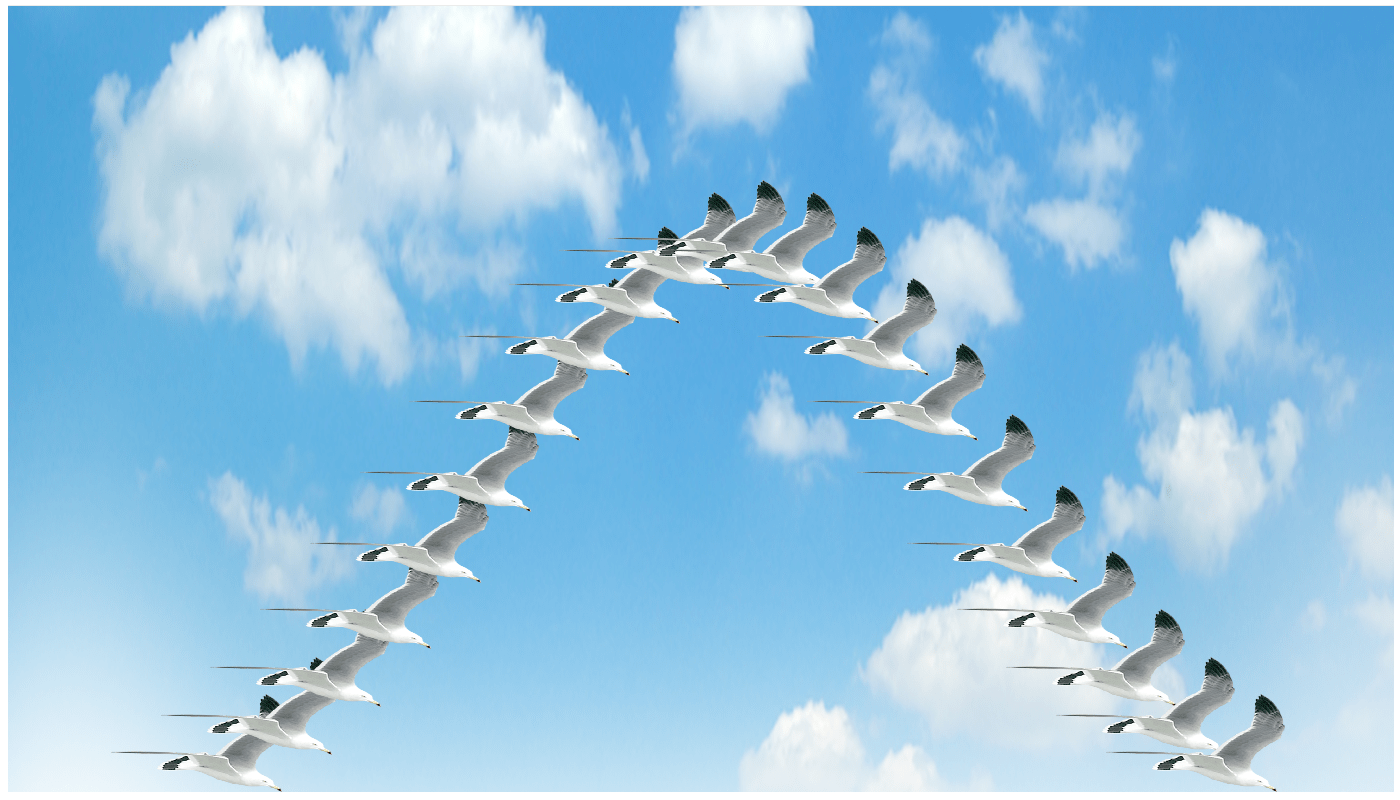Now I’m going to show you how to plot some data using one image as a marker and a different one for the back ground!

I will assume you have Rstudio installed and have at least a little experience with it but I’m aiming to make this do-able for beginners. I’ll also be using ggplot2 rather than base R.

### 1. Preparing

The first thing you want to do is make a folder on your computer in which the code you write and your images will live. Now you need some images.
I’m going to this as my backgroundand this as my marker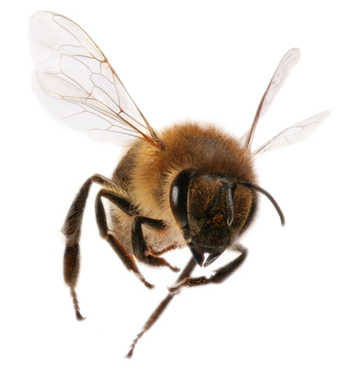You can use the same ones or use choose your own favourite images – try googling “public domain images”. You don’t want very high resolution images for the sake of speed but otherwise the size doesn’t matter.

The important thing is to save the images in to the folder you just created.

### 2. Getting started in RStudio

Start RStudio and set your working directory to the folder you created:Now start a new script file:and save it as funplot.R or similar.

There are several packages you will need. If your images files are png like mine: ggplot2, png, grid, ggimage.
If your images files are jpeg: ggplot2, jpeg, grid, ggimage.

All these are already installed for biologists at the University of York. If you are on your own computer you will need to install them.

Click the install button and write the package names in the box to install them. I recommending doing them one at a time.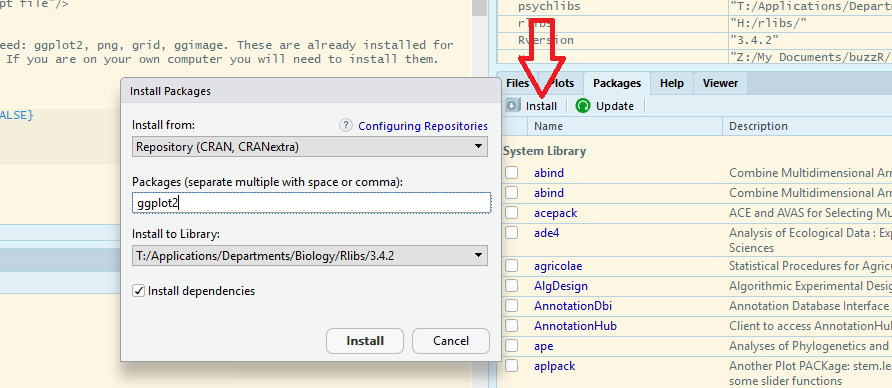Rstudio may need to install additional packages.

When everything is finished, you are ready to start coding!

### 3. Starting to code

Make the packages available for use in this Rstudio session by adding this code to your script and running it.

``````library(ggplot2)
library(png)
library(grid)
library(ggimage)
``````

### 4. Making some data to plot

I am going to plot the number of bees arriving within one hour at food sources placed at different distances from the hive. The data are as follows:

Distance (km) No.bees
0.5 40
1.0 34
1.5 31
2.0 22
2.5 18
3 10

To put these data in to a dataframe in R add this code to your script

``````bees <- data.frame(distance = c(0.5, 1, 1.5, 2, 2.5, 3),
number = c(40, 34, 32, 22,18, 10))
``````

Run that code. You do not need to re-run the first lines of code, just select the new lines and click run:### 5. A boring graph of the data 😦

A simple default can be obtained with:

``````ggplot(data = bees, aes(x = distance, y = number)) +
geom_point()
``````Edit that code to add information about the axis labels and axis limits

``````ggplot(data = bees, aes(x = distance, y = number)) +
geom_point() +
xlab("Distance (km)") +
ylab("Number of Bees") +
ylim(0, 45)
``````### 6. A fun graph of the data! 🙂

We need to read in the background file:

``````img <- readPNG("comb.png")
``````

Then we can edit the code with a `annotation_custom()` to add the background image:

``````ggplot(data = bees, aes(x = distance, y = number)) +
annotation_custom(rasterGrob(img,
width = unit(1,"npc"),
height = unit(1,"npc")),
-Inf, Inf, -Inf, Inf) +
geom_point() +
xlab("Distance (km)") +
ylab("Number of Bees") +
ylim(0, 45)
``````Make sure you put the `annotation_custom()` before the `geom_point()` but don’t worry too much about what the other bits mean.

To use bees as markers we need to add the bee image file name to every row of the data frame:

``````bees\$image <- "bee.png"
``````

This adds a column called image to the dataframe called bees. We don’t have to explicitly read the image in because the `ggimage` package we loaded takes care of that. `ggimage` is an ‘extension’ to ggplot2.

Now edit your graph code again by removing the `geom_point()` and adding a `geom_image()` instead like this:

``````ggplot(data = bees, aes(x = distance, y = number)) +
annotation_custom(rasterGrob(img,
width = unit(1,"npc"),
height = unit(1,"npc")),
-Inf, Inf, -Inf, Inf) +
geom_image(aes(image = image), size = 0.15) +
xlab("Distance (km)") +
ylab("Number of Bees") +
ylim(0, 45)
``````You can adjust the size of the marker by changing `size = 0.15` and get rid of the gray background (and add axes) with:

``````ggplot(data = bees, aes(x = distance, y = number)) +
annotation_custom(rasterGrob(img,
width = unit(1,"npc"),
height = unit(1,"npc")),
-Inf, Inf, -Inf, Inf) +
geom_image(aes(image = image), size = 0.15) +
xlab("Distance (km)") +
ylab("Number of Bees") +
ylim(0, 45) +
theme(panel.background = element_rect(fill = "white"))+
theme(axis.line.x = element_line(color = "black"),
axis.line.y = element_line(color = "black"))
``````Ta da!! Hope you enjoyed it and it was easy to follow.